﻿ Numerical Solution of the Total Probability Theorem in a Three Diensional Earthquake Source Domain for Developing Seismic<i> </i>Hazard Map and Hazard Spectrum

Numerical Solution of the Total Probability Theorem in a Three Diensional Earthquake Source Domain f...

Lalu Makrup, Masyhur Irsyam, I Wayan Sengara, Hendriyawan

Numerical Solution of the Total Probability Theorem in a Three Diensional Earthquake Source Domain for Developing SeismicHazard Map and Hazard Spectrum

Lalu Makrup1,, Masyhur Irsyam2, I Wayan Sengara2, Hendriyawan3

1Department of Civil Engineering, Islamic University of Indonesia, Yogyakarta

2Department of Civil Engineering, Institute of Technology Bandung

3Department of Ocean Engineering, Institute of Technology Bandung

Abstract

Probabilistic seismic hazard analysis is a method of assessing seismic threat on a region in the earth surface. Total probability theorem was utilized here has solved on earthquake source domain and employed it in seismic hazard analysis. Solution of the theorem is performed in a computer program and has been used to develop the Hazard Map and the Uniform Hazard Spectrum (UHS). The map is peak ground acceleration (PGA) of Sumatra Indonesia with hazard level 10% probability of exceedance in 50 years and the UHS is from a site in Surabaya Indonesia with hazard level 10% probability of exceedance in 50 years. The two result of the program are verified by the two previous study results. The result of the program is very similar to two other studies.

• Lalu Makrup, Masyhur Irsyam, I Wayan Sengara, Hendriyawan. Numerical Solution of the Total Probability Theorem in a Three Diensional Earthquake Source Domain for Developing Seismic Hazard Map and Hazard Spectrum. American Journal of Civil Engineering and Architecture. Vol. 3, No. 5, 2015, pp 158-164. http://pubs.sciepub.com/ajcea/3/5/2
• Makrup, Lalu, et al. "Numerical Solution of the Total Probability Theorem in a Three Diensional Earthquake Source Domain for Developing Seismic Hazard Map and Hazard Spectrum." American Journal of Civil Engineering and Architecture 3.5 (2015): 158-164.
• Makrup, L. , Irsyam, M. , Sengara, I. W. , & Hendriyawan. (2015). Numerical Solution of the Total Probability Theorem in a Three Diensional Earthquake Source Domain for Developing Seismic Hazard Map and Hazard Spectrum. American Journal of Civil Engineering and Architecture, 3(5), 158-164.
• Makrup, Lalu, Masyhur Irsyam, I Wayan Sengara, and H Hendriyawan. "Numerical Solution of the Total Probability Theorem in a Three Diensional Earthquake Source Domain for Developing Seismic Hazard Map and Hazard Spectrum." American Journal of Civil Engineering and Architecture 3, no. 5 (2015): 158-164.

 Import into BibTeX Import into EndNote Import into RefMan Import into RefWorks

123456
Prev Next

1. Introduction

Previously, methodology of probabilistic seismic hazard calculation was based on an assumption that seismic source as a point. Calculation was executed through a common statistical procedure that is a combination of regression technique and Gumbel standard probability distribution. Cornell  introduced new seismic source, it was area source zone. The source zone has been used as a basis to calculate seismic hazard. The area source is defined based on epicenter distribution of earthquakes that were occurred in a region (Figure 1).Download asVeiw figureFigures index
Figure 1. Seismic area source zone , used by permission

At this time, the earthquake source zone that was used as a basis to calculate seismic hazard are faults. The faults comprise subduction and transform fault source zone. As area source zones, these sources were defined also based on the epicenter distribution in their region. For earthquakes that were occurred on a region where the fault cannot be identified, their source is considered to area or background source .

Seismic hazard study has been performed  to develop Indonesian seismic hazard map. Irsyam et al.,  employed USGS analysis seismic hazard computer program in that study. The map is proposed for revising of Indonesian seismic building code. Generally, the study used the fault source zone. By employing USGS analysis seismic hazard program Petersen et al.,  developed seismic hazard map for Southeast Asia utilized the fault source zones. The Petersen study is funded as a USGS project.

In the present study I developed computer program to solve total probability theorem with earthquake source is fault. The two results of the program are verified by the two previous result study.

2. Total Probability Theorem

Probabilistic concept has allowed uncertainties in the site, location, and rate of recurrence of earthquake and in the variation of the ground motion characteristic with earthquake size and location to be explicitly considered in the evaluation of seismic hazards. Probabilistic Seismic Hazard Analysis (PSHA) provides a framework in which these uncertainties can be identified, quantified, and combined in rational manner to provide more complete picture of seismic hazard. For a given earthquake occurrence, the probability of a ground motion parameter A will exceed a particular value a can be computed using total probability theorem , that is,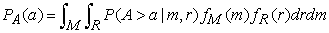(1)

where P(A>a|m,r) is a probability distribution of a particular value a will be exceeded a ground motion parameter A (the distribution was a log normal), fM(m) is a probability distribution of earthquake magnitude that commonly used is an exponential distribution which developed firstly by Gutenberg-Richter  fR(r) is a relative probability distribution of distance.

Equation (1) very difficult to solve analytically, even it almost cannot be solved analytically. Therefore the equation should be solved numerically. Commonly, each problem that has to be evaluated numerically need solution domain. The domain of this problem is an earthquake source (i.e. fault plain), see Figure 2.Download asVeiw figureFigures index
Figure 2. Fault plain in a three dimensional representation (drown based on McGuire, )

where:

A′B′AB = the plain where no energy release in this portion

ABCD = dipping fault plain

A′B′C′D′ = projection of fault plain on earth surface

EFGH = rupture area

EF = rupture length and EH = rupture width

E′F′G′H′ = projection of rupture area on horizontal plain

A′B′ = fault trace, TT′ = rupture portion on fault trace

EE′ = FF′ = top rupture depth

GG′ = HH′ = bottom rupture depth

2.1. Magnitude Probability Distribution

Magnitude distribution fM(m) is developed originated with Gutenberg-Richter’s law , equation (2).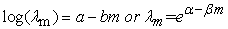(2)

where α ≈ 2.303a, β ≈ 2.303b, λm is number of event per year, a and b are regression constant that can be obtained by statistical procedure.

A truncated-below magnitude m0 can be introduced to the preceding formulation (equation 2) to exclude small magnitudes that can be ignored in engineering analysis. In mostly hazard analysis, m0 ranges between 3 and 5 . So, from equation (2) can be derived probability density function as: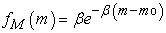(3)

Equation (3) allows probabilities calculation even for very large magnitude (i.e. unrealistic magnitude). To overcome this problem, an upper bound magnitude mu is introduced. It is defined as the largest earthquake likely to occur along an active source . Cornell and Vanmarcke  propose a modification to the original Gutenberg-Richter curve, accounting for mu as well as what has already accounted for m0. The actual value of mu should be determined by geological investigation of the region that will provide information about maximum fault rupture and therefore the maximum energy and magnitude that can be produced. The complete probability density function fM(m) for the magnitude range are expressed by: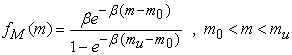(4)

The equation (4) is known as a truncated exponential distribution function (Figure 3).

Geologic and seismologic studies on a number of faults have shown that sources tend to repeat large earthquake that closes to their magnitude maximum, call characteristic earthquake. This was explained by observation that fault segment move by the same distance in each earthquake (constant fault slip rate).Download asVeiw figureFigures index
Figure 3. Exponential magnitude distribution (a) cumulative distribution function and (b) probability density function (drown based on equation 4)

The exponential model described in the previous paragraph is based on historical data solely and underestimate the rates of large earthquake as compare to geologic information. Youngs and Coppersmith  suggest an alternative recurrence law to account for the seismicity and rate of large event. Their model is called the characteristic earthquake recurrence law. By this approach, the cumulative distribution function flattens close to the maximum magnitude (Figure 4a). The probability density function that result from this model is a combination of truncated exponential Gutenberg-Richter model at small magnitude and uniformed distribution close to the maximum magnitude (Figure 4b).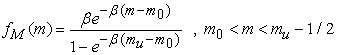(5)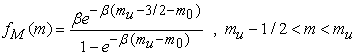(6)Download asVeiw figureFigures index
Figure 4. Characteristic magnitude distribution (a) cumulative distribution function and (b) probability density function 

The recurrence laws both Gutenberg-Richter  and Youngs and Coppersmith  are used in the program to describe the aleatory (or random) uncertainties in magnitude distribution.

2.2. Total Probability Theorem Solution and Distance Probability Distribution

A rupture and its magnitude can be occurred on different time and in everywhere on a fault plain. Thus probably occurrence of the rupture can be drawn as most of overlapped-rupture-area on whole of the fault plain (see Figure 5a). All equations related to the total probability theorem should be evaluated on fault plain (i.e. on each rupture area). Relative probability of a rupture that can be occurred on a fault will be the same as relative probability fR(r) of earthquake rupture-to-site distance.Download asVeiw figureFigures index
Figure 5. Likely occurrence of rupture on a fault plain (a) and definition of distance (b)

Rupture-to-site distance can be defined as in Figure 5b where:

RHIPO = Hypocenter distance (i.e. assumed hypocenter point is central of the rupture)

RJB = Joiner-Boore distance

REPI = Epicenter distance (i.e. projection of hypocenter point on the earth surface)

RRUP = Rupture distance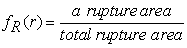(7)

The distances that are defined above can be evaluated if length and width of rupture were known. There are many relations that can be used to calculate rupture length. Well and Coppersmith  have given the formulation for shallow crustal event for all types of slip (equation 8).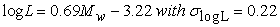(8)Download asVeiw figureFigures index
Figure 6. Probability distribution of distance

In PSHA the experts were commonly used the rupture width equal to rupture length . Thus for the same magnitude relate to equation (8) the rupture length and width are obtained equal to L. Based on above paragraph, then all events that likely will be occurred in the future on a fault with a magnitude value give flatten probability distribution (Figure 6).

2.3. Probability of Seismic Parameter to be Exceeded

Probability of a ground motion parameter A will exceed a particular value a, P(A>a|m,r), assuming log-normally distributed or logarithm of data are normally distributed (follow Gaussian distribution). According to probabilistic seismic hazard analysis, the standard normal deviate (z*) of the ground motion parameter is: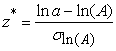(9)

where A is a ground motion parameter will exceed a particular value a. Probability of A exceed a P(A>a|m,r) = p(z*), see Figure 7, can be looked for in normal distribution table or it can be calculated using approximate formula :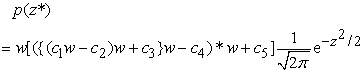(10)

where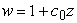(11)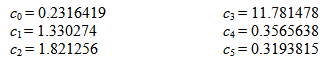3. Seismic Hazard Curve and Ground Motion Design

Frequency of a seismic event λ(A>a) for n number of earthquake sources was accounted for by the function: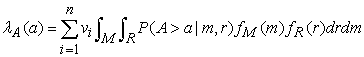(12)

where R is distance rupture-to-site, and M is magnitude.Download asVeiw figureFigures index
Figure 7. Probability diagram of P(a>A|M,R)Download asVeiw figureFigures index
Figure 8. Example of seismic hazard curve (a) and uniform hazard spectrum (b) (drown based on equation 13 and 14)

Relation between λ(A>a) and a, is called seismic hazard curve (Figure 8a). A combination of seismic hazard curve with Poisson distribution equation (13) will give ground motion design (Figure 8a).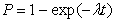(13)

According to ground motion prediction relation and its period (the attenuation function) so based on equation (12) and (13) can be accounted for a spectrum of ground motion parameter (e.g. acceleration ground motion) in probabilistic framework as a curve. The curve was called uniform hazard spectrum (Figure 8b).

4. Seismic Hazard Calculation and Program Verification

Seismic hazard calculation is executed by the program that is developed based on the theory above. This calculation is needed to acquire the hazard result that will be verified by other study. The two previous study results i.e. (1) Irsyam et al.,  developed seismic hazard map for Sumatra, and (2) Aldiamar,  developed uniform hazard spectrum for Surabaya Indonesia. The two results will be used to verify the result of the program.

To calculate the hazard Irsyam et al.,  used attenuation relations of Youngs et al.,  for subduction zone and Boore et al.,  and Sadigh et al.,  for shallow crustal event. In the other hand to calculate the hazard Aldiamar,  used Youngs et al.,  and Atkinson - Boore  for subduction zone and Boore – Atkinson , Campell – Bozorgnia , Chiou – Youngs  for shallow crustal event. Result of hazard computation by two authors can be seen in Figure 11 and Figure 12.Download asVeiw figureFigures index
Figure 9. Seismic source used by Irsyam et al.,Download asVeiw figureFigures index
Figure 10. Seismic source used by Aldiamar,Download asVeiw figureFigures index
Figure 11. Seismic hazard map (PGA) of Sumatra with hazard level 10% probability of exceedance in 50 years, developed by Irsyam et al., 

The two authors used hazard level 10% in 50 years as basis to calculate and employ EZ Frisk program  to compute the hazard.Download asVeiw figureFigures index
Figure 12. Uniform hazard spectrum of Surabaya with hazard level 10% probability of exceedance in 50 years, developed by AldiamarDownload asVeiw figureFigures index
Figure 13. Seismic hazard map (PGA) of Sumatra with hazard level 10% probability of exceedance in 50 years, developed in this study (2012)Download asVeiw figureFigures index
Figure 14. Uniform hazard spectrum of Surabaya with hazard level 10% probability of exceedance in 50 years, developed in this study

All of sources and sites, including seismic parameters used by two authors utilized to develop seismic hazard map of Sumatra and uniform hazard spectrum of Surabaya Indonesia respectively for verification purpose. Result of hazard calculation by the program of this study shown below in Figure 13 and Figure 14.

5. Comparison of Result

Comparison of the study results of Irsyam et al.,  and Aldiamar,  versus the study results is shown in Figure 12 and Figure 13.Download asVeiw figureFigures index
Figure 15. Comparison of PGA map of Sumatra with 10% probability in 50 years between Figure 9 and Figure 11

Based on those comparisons can be seen that the map of this study result very similar to that map of Irsyam et al.  study, and uniform hazard spectrum of this study result very similar to that uniform hazard spectrum of Aldiamar  study.Download asVeiw figureFigures index
Figure 16. Comparison of uniform hazard spectrum of Surabaya between Figure 12 and Figure 14

6. Conclusion

Computer program has been developed to calculate probabilistic seismic hazard. The program has been used to develop Sumatra seismic hazard map and ’s Uniform Hazard Spectrum (UHS). The result map and UHS has been compared to other studies. Those comparisons shown the map and UHS obtained from this study very similar to both of other studies respectively. Therefore the computer program can be employed for probabilistic seismic hazard analysis

References

  Aldiamar (2007). Analisis Risiko Gempa dan Pembuatan Respon Spektra untuk Jembatan Suramadu dengan Pemodelan Sumber Gempa Tiga Dimensi. Thesis. of , 2007.In article  Atkinson, G.M. and Boore, D.M. (2003). “Empirical ground-motion relations for subduction-zone earthquakes and their application to Cascadia and other regions”, Bulletin of the Seismological Society of America, v. 93, p. 1703-1729.In article View Article  Boore, D.M., and Atkinson G. M, (2006), Boore-Atkinson provisional NGA empirical ground-motion model for the average horizontal component of PGA, PGV and SA at spektral periods of 0.05, 0.1, 0.2, 0.3, 0.5, 1, 2, 3, 4, and 5 seconds, v 1.7 (October, 2006), http://peer.berkeley.edu/ products/Boore-Atkinson-NGA_11-13-06.html.In article  Campbell, K.W., Bozorgnia, Y., (2006), Campbell-Bozorgnia NGA Empirical Ground Motion Model for the Average Horizontal Component of PGA, PGV, PGD and SA at Selected Spektral Periods Ranging from 0.01-10.0 Seconds (Version1.1), http://peer.berkeley.edu/products/campbell bozorgnia_nga _report_ files/Campbell Bozorgnia NGA Report_DEC 15 2006_Ver1.1.In article  Chiou, B S.J.; Youngs, R.R, (2006). Chiou and Youngs PEER-NGA Empirical Ground Motion Model for the Average Horizontal Component of Peak Acceleration and Pseudo-Spektral Acceleration for Spektral Periods of 0.01 to 10 Seconds-Interim Report for USGS Review - June 14, 2006 (Revised Editorially July 10,2006), http://peer. Berkeley.edu/products/CYProgram/Chiou-Youngs-NGA, 2006.In article  Cornell, C.A. (1968): “Engineering Seismic Risk Analysis”, Bulletin of Seismological Society of America, Vol. 58, pp. 1583-1606.In article  Cornell, C.A. and Vanmarcke, E.H. (1969): “The Major Influences on Seismic Risk”, Proc. of the 4th World Conf. on Earthquake Engineering, Santiago, Chile.In article PubMed  Electric Power Research Institute (1986): “Seismic Hazard Methodology for the Central and Eastern United States”, EPRI, Palo Alto, EPRI, NP-4726.In article  Gutenberg, B. & Richter, C. F. (1944), Frequency of Earthquake in California, Bulletin of the Seismological Society of America, Vol.34, No. 4, pp. pp. 1985-1988.In article  Gardner, J.K., Knopoff, L., 1974. Is the sequence of earthquakes in southern California, with aftershocks removed, Poissonian? Bull. Seismol. Soc. Am. 64, 1363-1367.In article  Kramer S.L.( 1996), Geotechnical Earthquake Engineering. Prentice Hall:New Jersey.In article  Irsyama M, Asrurifaka M, Hendriyawana, Budionoa B, Triyoso W, and Firmanti A (2010). Development of spectral hazard maps for a proposed revision of the Indonesian Seismic Building Code. Geomechanics and Geoengineering: An International Journal, Vol. 5, No. 1, March 2010, 35-47.In article View Article  Irsyam M., Dangkua D.T., Hendriyawan, Hoedajanto D., Hutapea B. M., Kertapati E.K., Boen T., dan Petersen M. D, et al., (2008), The Proposed seismic hazard maps of Sumatra and Java islands and microzonation study of Jakarta city, Indonesia, J. Earth Syst. Sci. 117, S2, November 2008, pp. 865-878.In article View Article  McGuire R. K. (1976), EQRISK Evaluation of Sites for Earthquake Risk. United States Department of the Interior, Geological Survey, A Computer Program Distributed by NISEE/Computer Applications.In article  McGuire R. K. (2005), EZ Frisk Versions 7 manual, Risk Engineering Inc.In article  Nicolaou.A.S. (1998), A GIS Platform for Earthquake Risk Analysis. A dissertation submitted to the Faculty of the Graduate School of State University of New York at Buffalo USA in partial fulfillment of the requirement for the degree of Doctor of Philosophy, August.In article  Petersen, M.D., Dewey, J., Hartzell, S., Mueller, C., Harmsen, S., Frankel, A.D., and Rukstales, K., (2004), Probabilistic seismic hazard analysis for Sumatra, Indonesia and across the Southern Malaysian Peninsula. Elsevier.In article  Petersen, M.D., Harmsen, S., Mueller, C., Haller, K., Dewey, J., Luco, N., Crone, A., Rukstales, K., and Lidke, D., (2008), Probabilistic seismic hazard for Southeast Asia. International Conference of Earthquake Engineering and Disaster Mitigation, Jakarta, April 14, 15, 2008.In article  Sadigh K. et. al., (1997), Attenuation Relationships for shallow Crustal Earthquake Based on California Strong Motion Data, Seismological Research Letters, Volume 68 Januari/Pebruary 1997, Seismological Society of America.In article  Schwartz. D.P., and Coppersmith. K.J., (1984). “Fault behavior and characteristic earthquakes - Examples from the Wasatch and San Andreas fault zones”, Journal of Geophysical Research, v. 89, no. B7, p. 5681-5698.In article View Article  Wells. D.L., and Coppersmith. K.J., (1994). “New empirical relationships among magnitude, rupture length, rupture width, and surface displacements”, Bulletin of the Seismological Society of America, v. 84. p. 974-1002.In article  Youngs, R.R., Chiou, S. J, dan Silva W.J., (1997), Strong Ground Motion Attenuation Relationships for Subduction Zone Earthquakes, Seismological Research Letters, Volume 68 Januari/Pebruary 1997, Seismological Society of America.In article  Youngs, R.R. and Coppersmith, K.J. (1985): “Implications of Fault Slip Rates and earthquake Recurrence Models to Probabilistic Seismic Hazard Estimates”, Bulletin of the Seismological Society of America, Vol. 75, No.4, pp. 939-964.In article